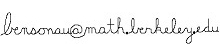# Benson Au

•Office:
• 747 Evans Hall

I am a sixth-year graduate student in the mathematics department at the University of California, Berkeley. I am interested in random matrices and related topics, particularly within the framework of non-commutative probability. My advisor is Steve Evans. I passed my qualifying exam on May 29th, 2014.

Research articles:
1. (with Guillaume Cébron, Antoine Dahlqvist, Franck Gabriel, and Camille Male) Large permutation invariant random matrices are asymptotically free over the diagonal. arXiv:1805.07045.
2. Traffic distributions of random band matrices. arXiv:1601.02188.
Teaching:
• Fall 2017: Math 16A (Analytic Geometry and Calculus), with Dr. Paulin.
• Spring 2017: Math 16B (Analytic Geometry and Calculus), with Dr. Paulin.
• Fall 2016: Math 110 (Linear Algebra), with Prof. Stankova.
• Fall 2015: Math 16A (Analytic Geometry and Calculus), with Dr. Paulin.
• Fall 2014: Math 53 (Multivariable Calculus), with Prof. Agol.
• Spring 2014: Math 16B (Analytic Geometry and Calculus), with Dr. Coward.
• Fall 2013: Math 16B (Analytic Geometry and Calculus), with Prof. Demmel.
• Spring 2013: Math 1B (Calculus), with Prof. Aganagic.
• Fall 2012: Math 1A (Calculus), with Prof. Borcherds.